JEE  >  JEE Main Previous year questions (2016-20): States of Matter

# JEE Main Previous year questions (2016-20): States of Matter - Notes | Study Chemistry for JEE - JEE

 1 Crore+ students have signed up on EduRev. Have you?

Q.1. The relative strength of interionic/intermolecular forces in decreasing order is    (2020)
(1) Dipole-dipole > ion-dipole > ion-ion
(2) Ion-dipole > ion-ion > dipole-dipole
(3) Ion-dipole > dipole-dipole > ion-ion
(4) Ion-ion > ion-dipole > dipole-dipole
Ans.
(4)
Since, ions have permanent charge on them. So their interaction is strongest and dipoles have partial charges on them. So their interactions are weaker than ions. Thus, the relative strength of the interionic / intermolecular interaction in decreasing order is: ion-ion > ion – dipole > dipole – dipole.

Q.2. Identify the correct labels of I, II and III in the following graph from the options given below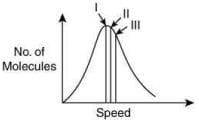Root mean square speed (Vrms); most probable speed (Vmp); Average speed (Vav)    (2020)
(1) I – Vmp; II – Vrms; III – Vav
(2) I – Vav; II – Vrms; III – Vmp
(3) I – Vrms; II – Vmp; III – Vav
(4) I – Vmp; II – Vav; III – Vrms
Ans.
(4)
The correct graphical representation of different speeds of the molecules and the number of molecules is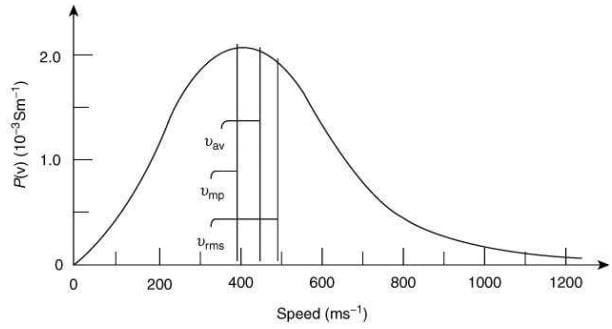Q.3. 0.5 moles of gas A and x moles of gas B exert a pressure of 200 Pa in a container of volume 10 m3 at 1000 K. Given R is the gas constant in JK-1 mol-1, x is:    (2019)
(1)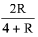(2)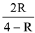(3)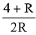(4)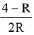Ans.
(4)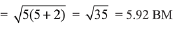Ideal gas equation: PV=nRT
After putting the values we get,
200 x 10 = (0.5 + x) x R x 1000
(total no. of moles are 0.5 + x)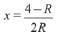Q.4. The volume of gas A is twice than that of gas B. The compressibility factor of gas A is thrice than that of gas B at same temperature. The pressures of the gases for equal number of moles are:    (2019)
(1) 3PA = 2PB
(2) 2PA = 3PB
(3) PA = 3PB
(4) PA = 2PB
Ans.
(2)
Compressibility factor is given by,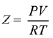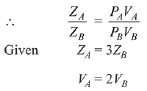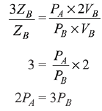Q.5. The combination of plots which does not represent isothermal expansion of an ideal gas is:    (2019)
(A)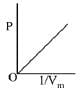(B)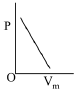(C)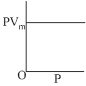(D)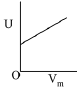(1) (B) and (D)
(2) (A) and (C)
(3) (B) and (C)
(4) (A) and (D)
Ans.
(1)
Isothermal expansion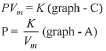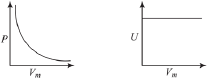Therefore (B) and (D) are not correct representation.

Q.6. An open vessel at 27°C is heated until two fifth of the air (assumed as an ideal gas) in it has escaped from the vessel. Assuming that the volume of the vessel remains constant, the temperature at which the vessel has been heated is:    (2019)
(1) 500°C
(2) 500 K
(3) 750°C
(4) 750 K
Ans.
(2)
At 27°C or 300 K number of moles of an ideal gas = n1. At T2 K number of moles of the ideal gas = n2
Number of moles escaped = 2n1/5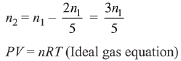At constant volume and pressure.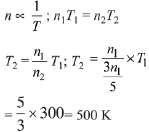Q.7. Consider the van der Waals constants, a and b, for the following gases,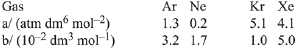Which gas is expected to have the highest critical temperature?    (2019)
(1) Kr
(2) Ne
(3) Xe
(4) Ar
Ans.
(1)
Critical temperature = 8a/27Rb
Value of a/b is highest for Kr. Therefore, Kr has greatest value of critical temperature.

Q.8. At a given temperature T, gases Ne, Ar, Xe and Kr are found to deviate from ideal gas behaviour. Their equation of state is given as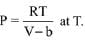Here, b is the van der Waals constant. Which gas will exhibit steepest increase in the plot of Z (compression factor) vs P?    (2019)
(1) Xe
(2) Kr
(3) Ne
(4) Ar
Ans.
(1)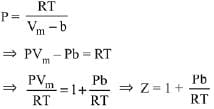[Where Z (compressibility factor) = PVm/RT]
Slope of Z vs P curve (straight line) = b/RT
∴ Higher the value of b, more steep will be the curve. Constant ‘b’ value depends on size of atom or molecule.

Q.9. Consider the following table: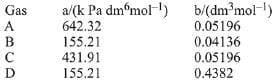a and b are vander waals constants. The correct statement about the gases is :    (2019)
(1) Gas C will occupy more volume than gas A; gas B will be more compressible than gas D
(2) Gas C will occupy lesser volume than gas A; gas B will be lesser compressible than gas D
(3) Gas C will occupy more volume than gas A; gas B will be lesser compressible than gas D
(4) Gas C will occupy lesser volume than gas A; gas B will be more compressible than gas D
Ans.
(1)
If values of 'b' for two gases are same but values of 'a' are different, then the gas having a larger value of 'a' will occupy lesser volume. Since, it, will have larger force of attraction and, therefore, lesser distance between its moleucles. If values of 'a' for two gases are same but values of 'b', are different then the smaller value of 'b' will occupy lesser volume and, therefore, will be more compressible.

Q.10. Points I, II and III in the following plot respectively correspond to (Vmp : most probable velocity)    (2019)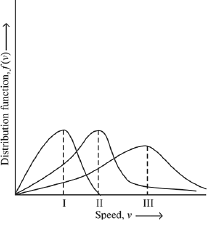(1) Vmp of N2 (300 K); Vmp of O2 (400 K); Vmp of H2 (300 K)
(2) Vmp of O2 (400 K); Vmp of N2 (300 K); Vmp of H2 (300 K)
(3) Vmp of N2 (300 K); Vmp of H2 (300 K); Vmp of O2 (400 K)
(4) Vmp of H2 (300 K); Vmp of N2 (300 K); Vmp of O2 (400 K)
Ans.
(1)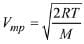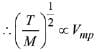From curve,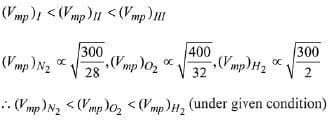Q.11. Assuming ideal gas behaviour, the ratio of density of ammonia to that of hydrogen chloride at same temperature and pressure is: (Atomic wt. of Cl 35.5 u)    (2018)
(1) 1.46
(2) 1.64
(3) 0.46
(4) 0.64
Ans.
(3)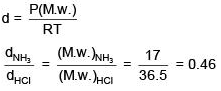Q.12. Among the following, the incorrect statement is:    (2017)
(1) At low pressure, real gases show ideal behaviour.
(2) At very low temperature, real gases show ideal behaviour.
(3) At Boyle's temperature, real gases shows idela behaviour.
(4) At very large volume, real gases show ideal behaviour.
Ans.
(2)

Q.13. An ideal gas undergoes isothermal expansion at constant pressure. During the process:    (2017)
(1) enthalpy remains constant but entropy increases.
(2) enthalpy decreases but entropy increases.
(3) enthalpy increases but entropy decreases.
(4) Both enthalpy and entropy remain constant.
Ans.
(1)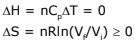Q.14. At very high pressures, the compressibility factor of one mole of a gas is given by:    (2016)
(1) 1 + pb/RT
(2) pb/RT
(3) 1 - b/(VRT)
(4) 1 - pb/RT
Ans.
(1)
According to Vander waal's equation for one mole of gas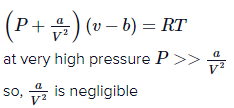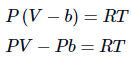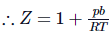Q.15. Two closed bulbs of equal volume (V) containing an ideal gas initially at pressure pi and temperature T1 are connected through a narrow tube of negligible volume as shown in the figure below. The temperature of one of the bulbs is then raised to T2. The final pressure Pf is:    (2016)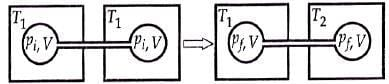(1)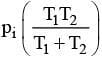(2)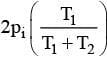(3)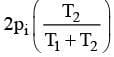(4)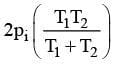Ans.
(3)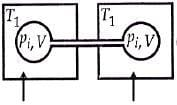n1 = piV/ RT1
n2 = piV/ RT1
Later on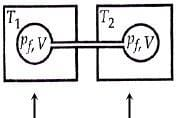n'1 = pfV/ RT1
n'2 = pfV/ RT2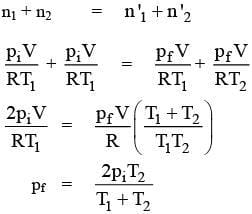Q.16. Initially, the root mean square (rms) velocity of N2 molecules at certain temperature is u. If this temperature is doubled and all the nitrogen molecules dissociate into nitrogen atoms, then the new rms velocity will be:    (2016)
(1) 2 u
(2) 14 u
(3) u/2
(4) 4 u
Ans.
(1)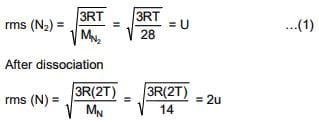The document JEE Main Previous year questions (2016-20): States of Matter - Notes | Study Chemistry for JEE - JEE is a part of the JEE Course Chemistry for JEE.
All you need of JEE at this link: JEE

## Chemistry for JEE

322 videos|586 docs|368 tests
 Use Code STAYHOME200 and get INR 200 additional OFF

## Chemistry for JEE

322 videos|586 docs|368 tests

Track your progress, build streaks, highlight & save important lessons and more!

,

,

,

,

,

,

,

,

,

,

,

,

,

,

,

,

,

,

,

,

,

;